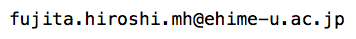Japanese pages are here.Hiroshi Fujita

Assistant Professor
Graduate School of Science and Engineering
Ehime University
E-mail:Published Papers

1. Hiroshi P. FUJITA, Mansfield and Solovay type results on covering plane sets by lines, Nagoya Mathematical Journal, vol.124 (1991), pages 145-155.
2. H. Fujita and S. Taniyama, On homogeneity of hyperspace of the Rationals, Tsukuba Journal of Mathematics, vol.20-1 (1996), pages 213--218.
3. H. Fujita, A measure theoretic basis theorem for $$\Pi^1_2$$, Journal of Mathematical Society of Japan, vol.52-2 (2000), pages 335--341.
4. Hiroshi Fujita and Dmitri B. Shakhmatov, Topological groups with dense compactly generated subgroups, Applied General Topology, vol.3-1 (2002), pages 85--89.
5. Hiroshi Fujita and Dmitri B. Shakhmatov, A characterization of compactly generated metric groups, Proceedings of American Mathematical Society, vol. 131-3 (2002), pages 953--961.
6. Hiroshi Fujita, Remarks on two problems by M.Laczkovich on functions with Borel measurable differences, Acta Mathematica Hungarica, vol. 117 (2007), pages 153--160.
7. Hiroshi Fujita and Tamás Tátrai, On difference property of Borel measurable functions, Fundamenta Mathematicae, vol. 208 (2010), pages 57--73.

Preprints

Abstract and link to the online resource

 A measure theoretic basis theorem for $$\Pi^1_2$$ [pdf]
If $$0^\sharp$$ exists, every $$\Pi^1_2$$ set of positive Lebesgue measure has a member that is arithmetically definable from $$0^\sharp$$. In this note we give a proof of this statement using unfolded covering game invented by Harrington and Kechris. Appears in Journal of the Mathematical Society of Japan.
 A weak basis theorem for $$\Pi^1_2$$ [pdf]
If $$\aleph_1^L$$ (the smallest ordinal which is uncountable in the constructible universe) is recursive in $$a\subset\omega$$, then every $$\Pi^1_2$$ set of positive Lebesgue measure has a member that is hyperarithmetically definable from $$a$$. This result gives an alternative proof of the main result of . It also suggests that the sort of basis result for $$\Pi^1_2$$ holds in univereses that are smaller than $$0^\sharp$$.
 Lebesgue's basis theorem [pdf]
As shown by H. Tanaka, every $$\Pi^0_1$$ set of positive Lebesgue measure contains an arithmetical real but it may fail to contain a recursive real. Here we prove, if A is a $$\Pi^0_1$$ set of reals and if the Lebesgue measure of A is a positive recursive real, then A contains a recursive real. The proof is based on H.Lebesgue's effective existence proof of normal numbers.
 Determinacy of covering games is equivalent to Lebesgue measurability [pdf]
Covering games has been invented by Leo Harrington in order to give a simplified proof of the Mycielski--Swierczkovski Theorem (that Axiom of determinacy implies Lebesgue measurability of every sets of reals). In fact the determinacy of covering games is a consequence of Lebesgue measurability, under ZF+DC.
 Remarks on two problems of Laczkovich on functions with Borel measurable differences [pdf]
(Published: Acta Mathematica Hungarica, vol. 117 (2007), pages 153--160.)
This note gives a partial answer to a problem raised by Miklós Laczkovich. Main result is: if $$f:{\mathbb R}\to{\mathbb R}$$ is Borel and the difference function $$\triangle_hf(x)$$ is of Baire class $$\alpha$$ for every $$h$$, then $$f$$ itself is of Baire class $$1+\alpha$$.
 On almost translation invariant sets of reals [pdf]
Some notes about sets of reals which are uncountable, co-uncountable and traslation invariant modulo countable sets. No Borel set has such property. It is independent of ZFC that such a $$\mathbf\Delta^1_2$$ set exists.
 A consistency result about functions with Borel measurable differences
If the existence of a measurable cardinal is consistent, so is the following statement: if $$f:{\mathbb R}\to{\mathbb R}$$ is bounded and the difference function $$\triangle_hf(x)$$ is Borel for every $$h$$, then $$f$$ itself is Borel. This means that in the construction of counter-example against Laczkovich's Problem 2 due to R.Filipów and I.Recław, the use of the Continuum Hypothesis is unavoidable.
(Added: Aug 22, 2008) This paper has been obsoleted by the next one.
 On difference property of Borel measurable functions [pdf]
(A joint paper with Tamás Mátrai. Published: Fundamenta Mathematicae 208(1), pp.57-73.)
If an atomlessly measurable cardinal exists, then the class of Lebesgue measurable functions, the class of Borel functions, and the Baire classes of all order have the difference property. This gives a consistent positive answer to Laczkovich's Problem 2. We also give a complete positive answer to Laczkovich's Problem 3 concerning Borel functions with Baire-α differences.

Seminar handouts etc.

 Coanalytic sets with Borel sections [pdf]
Seminar talk at 8th Kansai Set Theory Seminar (March 6 2009, Kobe University)
 On effectivization of Freiling's Axioms of Symmetry [pdf]
Proposes an alternative formulation of effective version of Axioms of Symmetry. Our version circumvents certain difficulties that Weitkamp's formulation possesses.
 On points of differentiability of discontinuous functions [pdf]
If $$A$$ and $$B$$ are disjoint $$F_\sigma$$ sets of real numbers, then there exists a real function which is discontinuous at each point of $$A$$, continous anywhere else, and differentiable at each point of $$B$$. If a real function is discontinous on a dense set, then its set of points of differentiability is meager. Based on a seminar talk given at Kobe University, Nov.18, 2010. Submitted to a forthcoming volume of RIMS Kokyuroku series.
 Notes on an old theorem of Erdös concerning CH [pdf]
An old theorem of Erdös says that the Continuum Hypothesis holds if and only if there exists an uncountable family $$\mathcal{F}$$ of entire functions on the complex plane whose section $$\{\,f(z)\,:\,f\in\mathcal{F}\,\}$$ is countable for every $$z$$. We show in this note that there exists such $$\mathcal{F}$$ among $$\Pi^1_1$$ sets if and only if every set of integers is constructible.

Some more?

There are several notes written in Japanese. Come here if you'd like to read Japanese text.